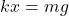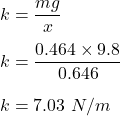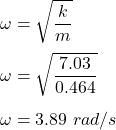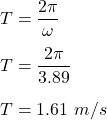## A block of mass 0.464 kg is hung from a vertical spring and allowed to reach equilibrium at rest. As a result, the spring is stretched by 0.

Question

A block of mass 0.464 kg is hung from a vertical spring and allowed to reach equilibrium at rest. As a result, the spring is stretched by 0.646 m. Find the spring constant. N/m The block is then pulled down an additional 0.338 m and released from rest. Assuming no damping, what is its period of oscillation? s How high above the point of release does the block reach as it oscillates?

in progress 0
2 months 2021-07-24T13:55:31+00:00 1 Answers 5 views 0

1. Explanation:

Mass, m = 0.464 kg

Compression in the spring, x = 0.646 m

(a) The net force acting on the spring is given by :k is spring constant(b) The angular frequency of the spring mass system is given by :The period of oscillation is :(c) As the spring oscillates, its will reach to a height of 2(0.338) = 0.676 m